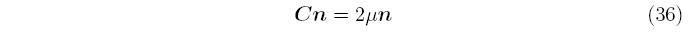# The continuum tangent matrix for plastic flow

Home » Knowledge Base » General » Materials » The continuum tangent matrix for plastic flow

Substituting Equations 33 and 20 into Equation 7 gives the continuum tangent matrix,For isotropic elasticity,which allows the elasto-plastic tangent matrix to be simplified towhere C is the standard linear isotropic elasticity matrix. This tangent is only used when there is plastic ow, and the elastic tangent is used otherwise.

djb 2005